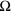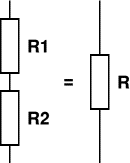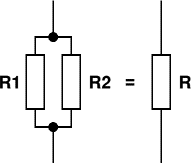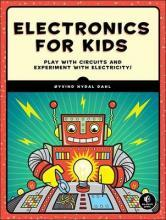# Resistance

Next Page: Ohm's Law

Also See: Resistors | Impedance

Resistance is the property of a component which restricts the flow of charge. Energy is used up as the voltage across the component drives current through it and this energy appears as heat in the component.

Resistance is measured in ohms, the symbol for ohm is an omega.

1is quite small for electronics so resistances are often given in kand M.

1 k= 10001 M= 1000000.

Resistors used in electronics can have resistances as low as 0.1or as high as 10M.

Rapid Electronics: Resistors

## Resistors connected in SeriesWhen resistors are connected in series their combined resistance is equal to the individual resistances added together. For example if resistors R1 and R2 are connected in series their combined resistance, R, is given by:

 Two resistors  in series: R = R1 + R2

This can be extended for more resistors:

 Resistors  in series: R = R1 + R2 + R3 + R4 + ...

The combined resistance in series will always be greater than any of the individual resistances.

## Resistors connected in ParallelWhen resistors are connected in parallel their combined resistance is less than any of the individual resistances.

There is a special equation for the combined resistance of two resistors R1 and R2 in parallel:

 Two resistors  in parallel: R = R1 × R2 R1 + R2

For more than two resistors connected in parallel a more difficult equation must be used. This adds up the reciprocal ('one over') of each resistance to give the reciprocal of the combined resistance, R:

 Resistors in parallel: 1 = 1 + 1 + 1 + ... R R1 R2 R3

The simpler equation for two resistors in parallel is much easier to use!

The combined resistance in parallel will always be less than any of the individual resistances.

## Conductors, Semiconductors and Insulators

The resistance of an object depends on its shape and the material from which it is made. For a given material, objects with a smaller cross-section or longer length will have a greater resistance.

Materials can be divided into three groups:

#### Conductors - low resistance

Examples include metals (aluminium, copper, silver etc.) and carbon. Metals are used to make connecting wires, switch contacts and lamp filaments. Resistors are made from carbon or long coils of thin wire.

#### Semiconductors - moderate resistance

Examples include germanium and silicon. Semiconductors are used to make diodes, LEDs, transistors and integrated circuits (chips).

#### Insulators - high resistance

Examples include most plastics such as polythene and PVC (polyvinyl chloride), paper, wood, rubber and glass. PVC is used as an outer covering for wires to prevent them making contact.

## Recommended bookI recommend Electronics for Kids as a good introduction to electricity and electronics. Printed in full colour with many illustrations, it introduces common components with simple but interesting projects to build at each stage. The book starts by assuming no previous knowledge then carefully builds up straightforward explanations of how components work, plus practical techniques including wire-stripping, soldering and using a multimeter.

Highlights include lighting an LED with lemons, using a relay to flash an LED, building a musical instrument, making a sunrise alarm, a colour-guessing game, a secret code checker and the final project uses three ICs to make a great game.

The author, Øyvind Nydal Dahl, has done a great job in providing clear step-by-step instructions with breadboard (or stripboard) layouts as well as circuit diagrams for projects. As Technical Reviewer for the book I've built all the projects myself and I'm very happy to recommend it to anyone looking for a fun and educational introduction to electronics.

Next Page: Ohm's Law | Study